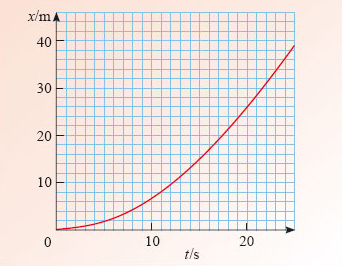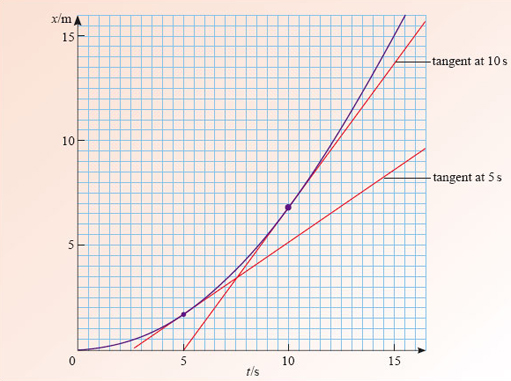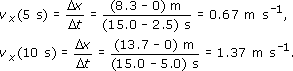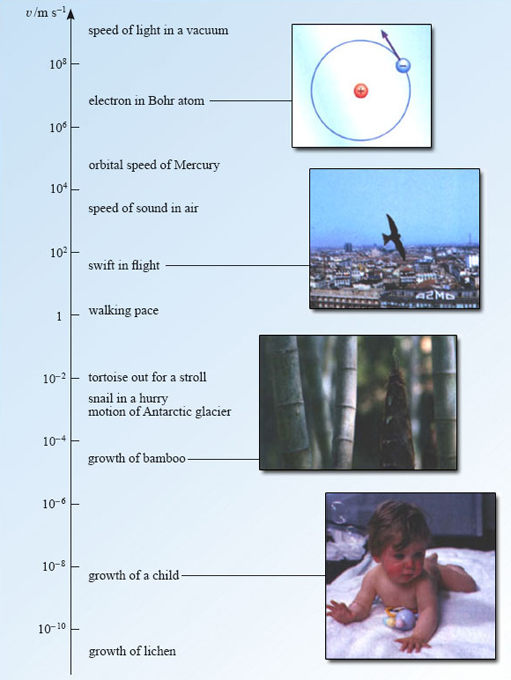Describing motion along a line

Start this free course now. Just create an account and sign in. Enrol and complete the course for a free statement of participation or digital badge if available.

Free course

# 4 Non-uniform motion along a line

## 4.1 Instantaneous velocity

Uniform motion is simple to describe, but is rarely achieved in practice. Most objects do not move at a precisely constant velocity. If you drop an apple it will fall downwards, but it will pick up speed as it does so (Figure 24), and if you drive along a straight road you are likely to encounter some traffic that will force you to vary your speed from time to time. For the most part, real motions are non-uniform motions.Figure 24: A falling apple provides an example of non-uniform motion. A sequence of pictures taken at equal intervals of time reveals the increasing speed of the apple as it falls

Figure 25 shows the position-time graph of an object that has an increasing velocity over the period t = 0 to t = 20 s; a car accelerating from rest. As you can see, the position-time graph is curved. There is relatively little change in position during the first few seconds of the motion but as the velocity increases the car is able to change its position by increasingly large amounts over a given interval of time. This is shown by the increasing steepness of the graph. In everyday language we would say that the graph has an increasing gradient, but you saw in the last section that the term gradient has a precise technical meaning in the context of straight-line graphs. Is it legitimate to extend this terminology to cover curved graphs, and if so, how exactly should it be done?Figure 25: The position-time graph for a car accelerating from rest

Extending the concept of gradient to the case of curved graphs is actually quite straightforward. The crucial point to recognise is that if you look closely enough at a small part of a smooth curve, then it generally becomes indistinguishable from a straight line. (In a similar way, the surface of the Earth is clearly curved when viewed from space, but each region is approximately flat when seen close-up.) So, if we choose a point on a curve we can usually draw a straight line passing through that point which has the same slope as the curve at the point of contact. This straight line is said to be the tangent to the curve at the point in question. Now, we already know how to determine the gradient of a straight line, so we can define the gradient at any point on a curve to be the gradient of the tangent to the curve at that point, provided the curve is sufficiently smooth that a tangent exists.

Figure 26 repeats the position-time graph of the accelerating car, but this time tangents have been added at t = 5 s and t = 10 s. The gradient of any such tangent represents a velocity and is referred to as the instantaneous velocity of the car at the relevant time. At least, that's what it should be called; in practice the word 'instantaneous' is often omitted, so references to 'velocity' should generally be taken to mean 'instantaneous velocity'. Allowing ourselves this informality, we can say:

velocity at time t = gradient of position-time graph at time t.

Although we have had to extend the meaning of gradient, we can still regard it as a measure of the rate of change of one variable with respect to another, so we can also say:

velocity at time t = rate of change of position with respect to time at time t.Figure 26: The instantaneous velocity at t = 5 s and at t = 10 s is determined by the gradient of the tangent to the position-time graph at each of those times

### Question 14

Estimate the (instantaneous) velocity of the car at t = 5 s and at t = 10 s (from Figure 26), and write down your answers taking care to distinguish one velocity from the other.

The relevant tangents are shown in Figure 26. Their gradients give the following estimates for the instantaneous velocitiesHow did you distinguish the velocity at t = 5 s from the velocity at t = 10 s? The conventional method is to use a common symbol for velocity, v﻿x, but to follow it by the relevant value of time enclosed in parentheses, as in v﻿x﻿(﻿5 s﻿) and v﻿﻿x﻿(﻿10 s﻿). This notation can also be used to indicate the velocity at any time t, by writing v﻿﻿x(t), even if the value of t is unspecified.

It is important to remember that v﻿x﻿(﻿t﻿) represents the (instantaneous) velocity at time t. It does not mean v﻿﻿x multiplied by t.

You will not be surprised to learn that the positive quantity |﻿v﻿x﻿(﻿t﻿)﻿| representing the magnitude of the instantaneous velocity at time t is called the instantaneous speed at time t. If we denote this by v﻿(﻿t﻿), we can writeSpeed and velocity are measured in the same units, m s−1. Some typical values of physically interesting speeds are listed in Figure 27.Figure 27: Some physically interesting speeds
S207_2